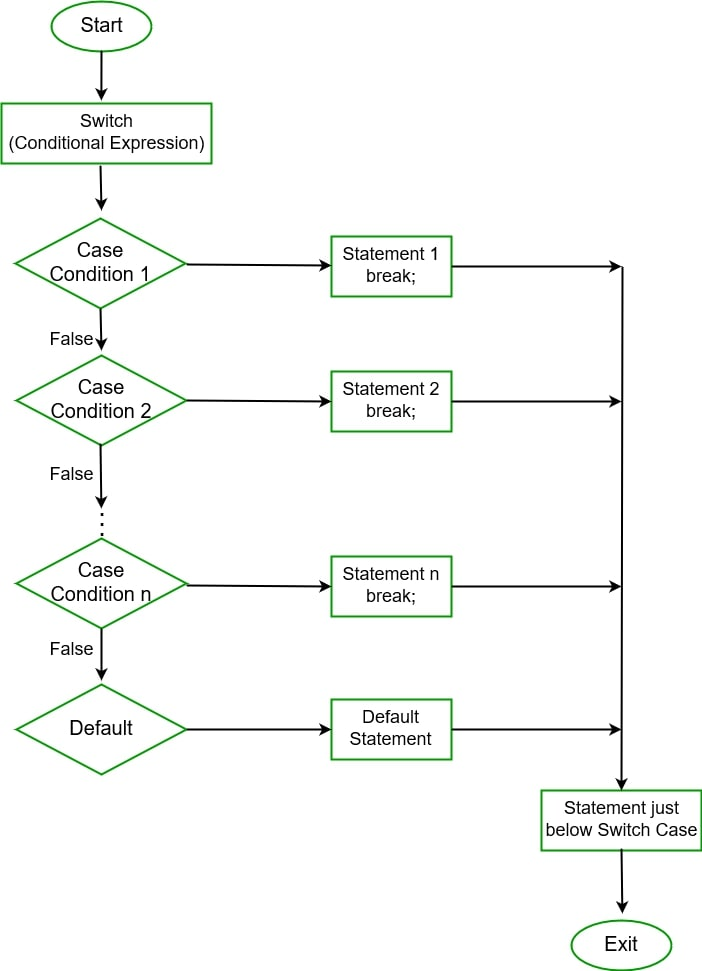# Switch Statement in Java

• Difficulty Level : Easy
• Last Updated : 25 Jun, 2022

The switch statement is a multi-way branch statement. In simple words, the Java switch statement executes one statement from multiple conditions. It is like an if-else-if ladder statement. It provides an easy way to dispatch execution to different parts of code based on the value of the expression. Basically, the expression can be a byte, short, char, and int primitive data types. It basically tests the equality of variables against multiple values.

Note: Java switch expression must be of byte, short, int, long(with its Wrapper type), enums and string. Beginning with JDK7, it also works with enumerated types (Enums in java), the String class, and Wrapper classes.### Some Important Rules for Switch Statements

1. There can be any number of cases just imposing condition check but remember duplicate case/s values are not allowed.
2. The value for a case must be of the same data type as the variable in the switch.
3. The value for a case must be constant or literal. Variables are not allowed.
4. The break statement is used inside the switch to terminate a statement sequence.
5. The break statement is optional. If omitted, execution will continue on into the next case.
6. The default statement is optional and can appear anywhere inside the switch block. In case, if it is not at the end, then a break statement must be kept after the default statement to omit the execution of the next case statement.

### Flow Diagram of Switch-Case StatementSyntax: Switch-case

```// switch statement
switch(expression)
{
// case statements
// values must be of same type of expression
case value1 :
// Statements
break; // break is optional

case value2 :
// Statements
break; // break is optional

// We can have any number of case statements
// below is default statement, used when none of the cases is true.
// No break is needed in the default case.
default :
// Statements
}```

Note: Java switch statement is a fall through statement that means it executes all statements if break keyword is not used, so it is highly essential to use break keyword inside each case.

Example: Consider the following java program, it declares an int named day whose value represents a day(1-7). The code displays the name of the day, based on the value of the day, using the switch statement.

## Java

 `// Java program to Demonstrate Switch Case``// with Primitive(int) Data Type`` ` `// Class``public` `class` `GFG {`` ` `    ``// Main driver method``    ``public` `static` `void` `main(String[] args)``    ``{``        ``int` `day = ``5``;``        ``String dayString;`` ` `        ``// Switch statement with int data type``        ``switch` `(day) {`` ` `        ``// Case``        ``case` `1``:``            ``dayString = ``"Monday"``;``            ``break``;`` ` `        ``// Case``        ``case` `2``:``            ``dayString = ``"Tuesday"``;``            ``break``;`` ` `            ``// Case``        ``case` `3``:``            ``dayString = ``"Wednesday"``;``            ``break``;`` ` `            ``// Case``        ``case` `4``:``            ``dayString = ``"Thursday"``;``            ``break``;`` ` `        ``// Case``        ``case` `5``:``            ``dayString = ``"Friday"``;``            ``break``;`` ` `            ``// Case``        ``case` `6``:``            ``dayString = ``"Saturday"``;``            ``break``;`` ` `            ``// Case``        ``case` `7``:``            ``dayString = ``"Sunday"``;``            ``break``;`` ` `        ``// Default case``        ``default``:``            ``dayString = ``"Invalid day"``;``        ``}``        ``System.out.println(dayString);``    ``}``}`

Output

`Friday`

### Omitting the break Statement

A break statement is optional. If we omit the break, execution will continue on into the next case. It is sometimes desirable to have multiple cases without break statements between them. For instance, let us consider the updated version of the above program, it also displays whether a day is a weekday or a weekend day.

Example:

## Java

 `// Java Program to Demonstrate Switch Case``// with Multiple Cases Without Break Statements`` ` `// Class``public` `class` `GFG {`` ` `    ``// main driver method``    ``public` `static` `void` `main(String[] args)``    ``{``        ``int` `day = ``2``;``        ``String dayType;``        ``String dayString;`` ` `        ``// Switch case``        ``switch` `(day) {`` ` `        ``// Case``        ``case` `1``:``            ``dayString = ``"Monday"``;``            ``break``;`` ` `        ``// Case``        ``case` `2``:``            ``dayString = ``"Tuesday"``;``            ``break``;`` ` `            ``// Case``        ``case` `3``:``            ``dayString = ``"Wednesday"``;``            ``break``;``        ``case` `4``:``            ``dayString = ``"Thursday"``;``            ``break``;``        ``case` `5``:``            ``dayString = ``"Friday"``;``            ``break``;``        ``case` `6``:``            ``dayString = ``"Saturday"``;``            ``break``;``        ``case` `7``:``            ``dayString = ``"Sunday"``;``            ``break``;``        ``default``:``            ``dayString = ``"Invalid day"``;``        ``}`` ` `        ``switch` `(day) {``            ``// Multiple cases without break statements`` ` `        ``case` `1``:``        ``case` `2``:``        ``case` `3``:``        ``case` `4``:``        ``case` `5``:``            ``dayType = ``"Weekday"``;``            ``break``;``        ``case` `6``:``        ``case` `7``:``            ``dayType = ``"Weekend"``;``            ``break``;`` ` `        ``default``:``            ``dayType = ``"Invalid daytype"``;``        ``}`` ` `        ``System.out.println(dayString + ``" is a "` `+ dayType);``    ``}``}`

Output

`Tuesday is a Weekday`

### Nested Switch Case statements

We can use a switch as part of the statement sequence of an outer switch. This is called a nested switch. Since a switch statement defines its own block, no conflicts arise between the case constants in the inner switch and those in the outer switch.

Example:

## Java

 `// Java Program to Demonstrate``// Nested Switch Case Statement`` ` `// Class``public` `class` `GFG {`` ` `    ``// Main driver method``    ``public` `static` `void` `main(String[] args)``    ``{``        ``// Custom input string``        ``String Branch = ``"CSE"``;``        ``int` `year = ``2``;`` ` `        ``// Switch case``        ``switch` `(year) {`` ` `        ``// Case``        ``case` `1``:``            ``System.out.println(``                ``"elective courses : Advance english, Algebra"``);`` ` `            ``// Break statement to hault execution here``            ``// itself if case is matched``            ``break``;`` ` `            ``// Case``        ``case` `2``:`` ` `            ``// Switch inside a switch``            ``// Nested Switch``            ``switch` `(Branch) {`` ` `            ``// Nested case``            ``case` `"CSE"``:``            ``case` `"CCE"``:``                ``System.out.println(``                    ``"elective courses : Machine Learning, Big Data"``);``                ``break``;`` ` `            ``// Case``            ``case` `"ECE"``:``                ``System.out.println(``                    ``"elective courses : Antenna Engineering"``);``                ``break``;`` ` `                ``// default case``                ``// It will execute if above cases does not``                ``// execute``            ``default``:`` ` `                ``// Print statement``                ``System.out.println(``                    ``"Elective courses : Optimization"``);``            ``}``        ``}``    ``}``}`

Output

`elective courses : Machine Learning, Big Data`

Example:

## Java

 `// Java Program to Illustrate Use of Enum``// in Switch Statement`` ` `// Class``public` `class` `GFG {`` ` `    ``// Enum``    ``public` `enum` `Day { Sun, Mon, Tue, Wed, Thu, Fri, Sat }`` ` `    ``// Main driver method``    ``public` `static` `void` `main(String args[])``    ``{`` ` `        ``// Enum``        ``Day[] DayNow = Day.values();`` ` `        ``// Iterating using for each loop``        ``for` `(Day Now : DayNow) {`` ` `            ``// Switch case``            ``switch` `(Now) {`` ` `            ``// Case 1``            ``case` `Sun:``                ``System.out.println(``"Sunday"``);`` ` `                ``// break statement that hault further``                ``// execution once case is satisfied``                ``break``;`` ` `            ``// Case 2``            ``case` `Mon:``                ``System.out.println(``"Monday"``);``                ``break``;`` ` `            ``// Case 3``            ``case` `Tue:``                ``System.out.println(``"Tuesday"``);``                ``break``;`` ` `            ``// Case 4``            ``case` `Wed:``                ``System.out.println(``"Wednesday"``);``                ``break``;`` ` `            ``// Case 5``            ``case` `Thu:``                ``System.out.println(``"Thursday"``);``                ``break``;`` ` `            ``// Case 6``            ``case` `Fri:``                ``System.out.println(``"Friday"``);``                ``break``;`` ` `            ``// Case 7``            ``case` `Sat:``                ``System.out.println(``"Saturday"``);``                ``break``;``            ``}``        ``}``    ``}``}`

Output

```Sunday
Monday
Tuesday
Wednesday
Thursday
Friday
Saturday```